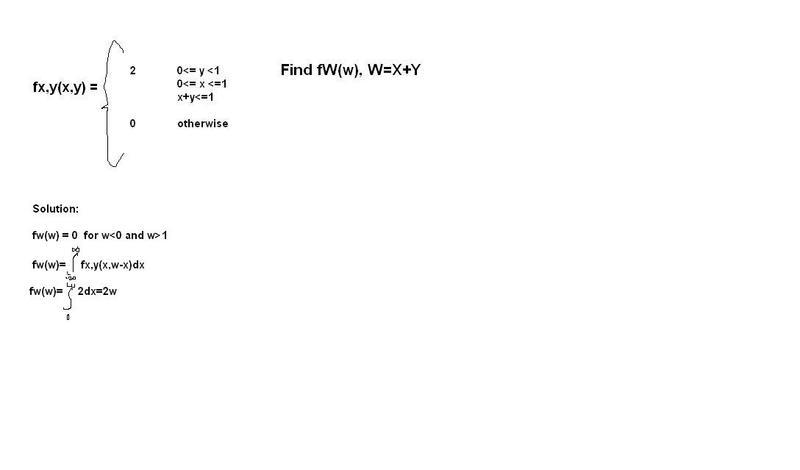# Pdf of sum of two random variables problem

Hi, everybody.

My problem is about Probability and Random Process.

i can't understand the probability density function of sum of two random variables and function of product of two random variables.

Here is my question with a part of a solution:

how can i find these problems solutions and where can i study on the internet ?